Conformal-differential geometry

(diff) ← Older revision | Latest revision (diff) | Newer revision → (diff)

A branch of conformal geometry in which the geometric quantities that are invariant under conformal transformations are studied by the methods of analysis, in the first instance, differential calculus.

In the conformal plane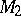each point or circle is defined by a vector, where the,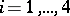, are the so-called tetracyclic coordinates. For a point one has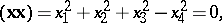and for a circle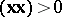. The conformal-differential geometry in the plane studies sequences and congruences of circles. To a sequence of circles corresponds a curve in three-dimensional hyperbolic space and to a congruence of circles — a surface. A sequence is given by a parametrization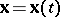. The parametercan be specialized to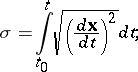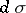being the angle between two infinitesimally-close circles in the sequence. Of special significance in the theory of sequences are the two branches of the envelope of this sequence,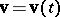and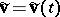, their osculating circles. As in ordinary differential geometry of curves, one can write derivation formulas for a sequence of circles by decomposing the derivatives of the vectors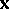,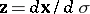,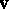,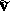in terms of themselves: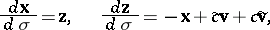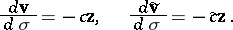Two invariantsandcan be obtained. The invariantis expressed in terms of the anglebetween the osculating circles of the envelope: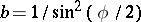. The theory of curves in the conformal plane is constructed from the theory of sequences of circles: each curve is regarded as an envelope, namely the sequence with invariant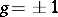. If, furthermore, the invariantis constant, then the curve turns out to be the isogonal trajectory of a pencil of circles, that is, a loxodrome.

In the three-dimensional spacethe equationdefines a sequence of spheres. In its study its enveloping surface, the so-called canal surface, plays an important role. Each sequence of spheres is characterized by three invariants, which are expressed in terms of certain angles determined by the spheres of the sequence.

A congruence of circles inis given by a parametrization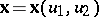. On the surface corresponding to it in the hyperbolic space, the polar normalization is conveniently introduced by taking as the normal of the first kind the line orthogonal to the tangent plane of the surface at the point, and as the normal of the second kind, the polar of the normal of the first kind with respect to the absolute(see ). In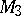the normalization of a congruence corresponds to the normalization of the surface: Associated with each circleis the circleorthogonal toand to each infinitesimally-close circle, and two circles, defining the pencil of the circles, conjugate to the pencil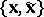:. Oninvariants of the congruence of circles are defined corresponding to the invariants of the associated surface in hyperbolic space, and special types of congruences are singled out.

In the theory of surfaces ina framing of the surface is introduced by means of normal circles orthogonal at each point to all tangent spheres of the surface; associated with each point is a conformal frame consisting of a point of the surface, two coordinate spheres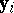,, defining normal circles, a tangent sphereat, and the point of intersectionof this sphere and the normal circle. In the general theory of normalization of surfaces one uses the isomorphism of the theory of normalized surfaces of a conformal space and the theory of interior polar normalizations of the absolute of a hyperbolic space. The interior geometry of a normalized surface inis a Weyl geometry whose first fundamental tensor is the same as the tensor of the angular metric of the surface, while the second fundamental tensor is the normalizer defining the supporting coordinates of the sphere.

The results obtained for a normalized surface are valid also for a normalized conformal space.

How to Cite This Entry:
Conformal-differential geometry. Encyclopedia of Mathematics. URL: http://encyclopediaofmath.org/index.php?title=Conformal-differential_geometry&oldid=12839
This article was adapted from an original article by G.V. Bushmanova (originator), which appeared in Encyclopedia of Mathematics - ISBN 1402006098. See original article